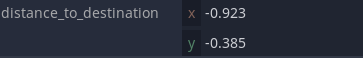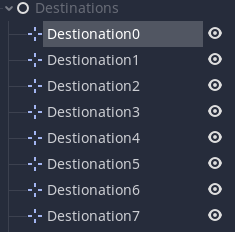+1 vote

Getting 2 errors
1. Invalid get index '0' (on base: 'PoolVector2Array').
2. Invalid operands 'Vector2' and 'int' in operator '>'.

``````extends "res://NPC/PlayerDetection.gd"

var motion
var possible_destinations
var path

export var minimum_arrival_distance = 5
export var walks_peed = 0.5

randomize()
possible_destinations = destinations.get_children()
make_path()

func _physics_process(delta):
navigate()

func navigate():
var distance_to_destination = position.direction_to(path)
if distance_to_destination > minimum_arrival_distance:
move()
else:
update_path()

func move():
look_at(path)
motion = (path - position).normalized() * (MAX_SPEED * walks_peed)

if is_on_wall():
make_path()

move_and_slide(motion)

func update_path():
if path.size() == 1:
if \$Timer.is_stopped():
\$Timer.start()
else:
path.remove(0)

func make_path():
var new_destination = possible_destinations[randi() % possible_destinations.size() - 1]

func _on_Timer_timeout():
make_path()
``````in Engine

+1 vote

For this one...

`Invalid get index '0' (on base: 'PoolVector2Array')`

I assume your array doesn't contain any elements at the time you make that call. If that's the case, I assume that the `get_simple_path` call isn't returning anything. Try printing out the number of elements in the array and see what you get (the `size()` function).

For this...

`Invalid operands 'Vector2' and 'int' in operator '>'`

The call to `direction_to` returns a `Vector2` while `distance_to_destination` is just a simple, scalar value. You can't compare the two different value types. I'm not sure what you want there, probably either a single component of the `Vector2` (so, it's X or Y component), or maybe the length of the `Vector2`?

by (12,286 points)

path return empty. Sometime it will work

destinationsSomeone else found the solution to this very issue, so I will share with you here.

Replace `direction_to` with `distance_to`.
Why? `distance_to` returns a float while `direction_to` returns a Vector2.

`distance_to_destination = position.direction_to(path)` returns a Vector2. `minimum_arrival_distance = 5` returns a int of 5. You can't compare Vector2 with Ints/Floats. It will lead to the game crashing.

The issue is related to this function:

``````func navigate():
var distance_to_destination = position.direction_to(path)
if distance_to_destination > minimum_arrival_distance:
move()
else:
update_path()
``````

Before finding this solution, I tried an earlier solution with limited success. It was instead of replacing `direction_to` with `distance_to`, just replace the `minimum_arrival_distance = 5` with `minimum_arrival_distance = Vector2()`. This will mean we are comparing two Vector2. That will allow the game to run without crashing and also allow the guards to move around. But their movement is buggy and they all end up getting stuck in one destination node after moving about for about a minute or so.

So this is the reason why instead, have both `distance_to_destination > minimum_arrival_distance` as floats as oppose to Vector2s.

by (14 points)
edited